## Inverse Cosine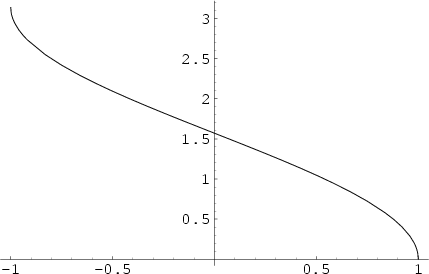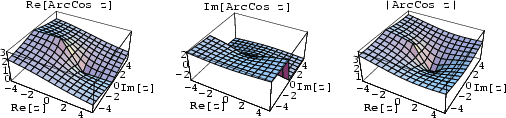The function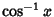, also denoted arccos(), where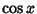is the Cosine and the superscript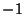denotes an Inverse Function, not the multiplicative inverse. The Maclaurin Series for the inverse cosine range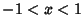is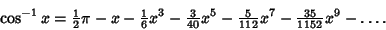(1)

The inverse cosine satisfies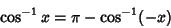(2)

for Positive and Negative, and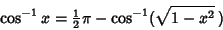(3)

for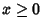. The inverse cosine is given in terms of other inverse trigonometric functions by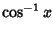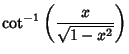(4)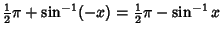(5)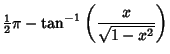(6)

for Positive or Negative, and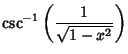(7)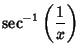(8)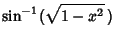(9)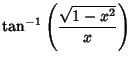(10)

for.

Abramowitz, M. and Stegun, C. A. (Eds.). Inverse Circular Functions.'' §4.4 in Handbook of Mathematical Functions with Formulas, Graphs, and Mathematical Tables, 9th printing. New York: Dover, pp. 79-83, 1972.